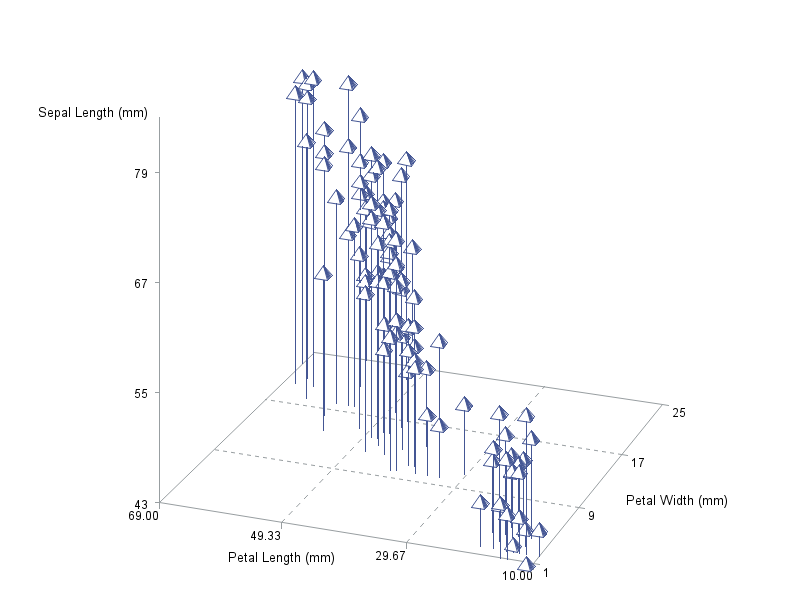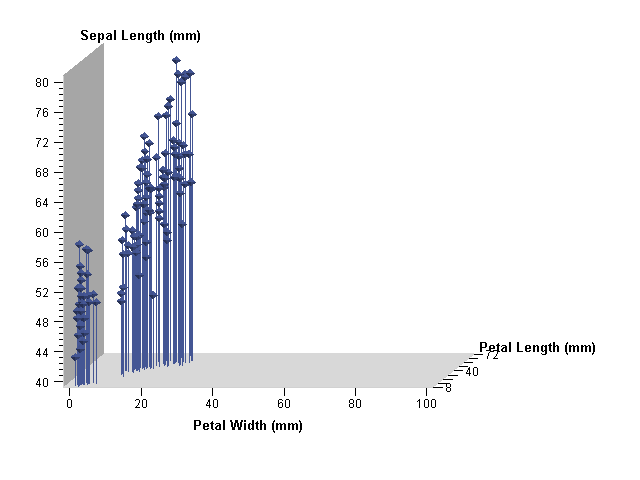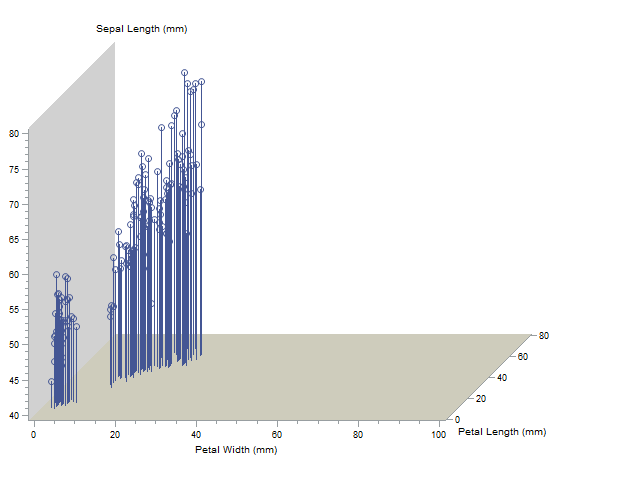## Proc g3d, controlling the x and y axes?

Hello all,

Is there a *simple* way of controlling the range and tickmarks for the x and y axes in proc g3d, or another procedure that may be easier to use?

I want to produce a 3D scatter graph from ordination data. In the past I created a dummy dataset with the maximum and minimum values for the axes and then merged this dataset with the actually data for graphing. However, SAS creates default values for the range on the x and y axes and the values for the tickmarks based on the data itself. For some reason (?) you can control the z-axis using zmax, zmin, and ztickmarks statements in the scatter <options> statement. I did look online and found a long and convoluted way of resetting the graphics defaults using a goptions statement, but was hoping someone out there has had a similar problem and has managed to figure out a simpler solution.

Thanks,

Bugmeister

4 REPLIES 4

## Re: Proc g3d, controlling the x and y axes?

OK, I think I have figured out the answer on my own, based on SAS support page. Here is the program with notes:

/* resets the graphics options, including the axes range and number of tickmarks for the grid */

goptions reset=all cback=white border htitle=12pt htext=10pt;

%let x_min=-2;

%let x_max=2;

%let x_ticks=3;

%let y_min=-2;

%let y_max=2;

%let y_ticks=3;

%let z_min=-2;

%let z_max=2;

%let z_ticks=3;

data one;

set [sas dataset name];

/* sets the options (symbol size, symbol type, symbol color) for the data points by variable */
if Origin = 'x' then do; symsize=0.5; end;

if Origin = 'y' then do; symsize=0.5; end;

if Age= '1' then do; symbol='flag'; end;

if Age= '15' then do; symbol='Balloon'; end;

if Age= '30' then do; symbol='Square'; end;

if Age= '70' then do; symbol='cross'; end;

if Age= '130' then do; symbol='Diamond'; end;

if Origin = 'x' then do; symcolor = 'red'; end;

if Origin = 'y' then do; symcolor = 'black'; end;

/* This next dataset creates points at the max and min for the axes, and then plots the data

I have three variables, Ax3 (x), Ax2 (y) and Ax1 (z) to plot */
data two;

set one end=last;

output;

if last;

Ax3=&x_min;

Ax2=&y_min;

Ax1=&z_min;

output;
Ax3=&x_max;

Ax2=&y_max;

Ax1=&z_max;

output;

run;

/* the above adds dummy variables at the max and min values for each axis, below plots the graph */

proc g3d;

scatter Ax3*Ax2=Ax1 /

grid

xticknum=&x_ticks

yticknum=&y_ticks

zticknum=&z_ticks

shape= symbol

size=symsize

color=symcolor

noneedle

rotate= -45;

label Ax2 = 'NMS Axis 2;

run;

## Re: Proc g3d, controlling the x and y axes?

Tickmark locations and labels for axis values for G3D should be using AXIS statements.

You define an Axis statement (you can have Axis1 to Axis99) prior to the prior to the procedure the assign the characteristics to the appropriate x, y or z axis using an option on the plot statement:

plot y*x=z / xaxis=Axis1 yaxis=axis2 zaxis= axis3;

where Axis1 might look like:

Axis1 label="Text for the axis label"

order = (0 to 100 by 10) /* to create tickmarks at 0, 10, 20, etc to 100*/

;

You can also do lots of things with AXIS statements.

## Re: Proc g3d, controlling the x and y axes?

Thanks bugmeister for posting this!  Why SAS help says to use axis statements I don't know.   G3D does not support them, as I just discovered.  Thanks for the workaround.

## Re: Proc g3d, controlling the x and y axes?

I don't use the g3d procedure much (because I avoid 3d graphs in general), but if I recall correctly, the standard implementation of proc g3d (device=png, gif, etc) does not allow you to control it with the axis statement.

The java and activex implementation provide partial support of the axis statement.

For example:

This one ignores the axis statement...

goptions device=png;

axis1 order=(0 to 100 by 20);

proc g3d data=sashelp.iris;

scatter PetalLength*PetalWidth=SepalLength / xaxis=axis1;

run;Whereas the java implementation partially supports it:

goptions device=javaimg;

axis1 order=(0 to 100 by 20);

proc g3d data=sashelp.iris;

scatter PetalLength*PetalWidth=SepalLength / xaxis=axis1;

run;And the activex implementation partially supports it:

goptions device=actximg;

axis1 order=(0 to 100 by 20);

proc g3d data=sashelp.iris;

scatter PetalLength*PetalWidth=SepalLength / xaxis=axis1;

run;Here's how the documentation words it:

SAS/GRAPH(R) 9.4: Reference, Third Edition

 Restriction: The AXIS statement is partially supported by Java and ActiveX devices only.
Discussion stats
• 4 replies
• 3172 views
• 0 likes
• 4 in conversation